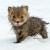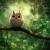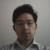## C语言，是否阶乘之和？

Apria 发布于 2017/02/19 20:34

`输入一个整数N，判断其是否可以表示成一个正整数阶乘的形式或者几个不同正整数的阶乘之和。`
`输入一个整数N。`
`对应该整数N，若可以表示，输出YES，否则输出NO`
```4
-1
0
6```
```NO
NO
NO
YES```

000``````// 判断整数n是否为一个或多个不同整数的阶乘和，如果是则返回1，如果不是则返回0。
int isFactorialSum(int n) {
if (n <= 0) {
return 0;
}

if (n == 1 || n == 2) {
return 1;
}

if (n % 2 == 1) {
return isFactorialSum(n - 1);
}

int k = 2;
while (1) {
if (n % k != 0) {
break;
}

while (n % k == 0) {
n /= k;
k++;
}

if (n == 1) {
return 1;
}

if (n < k) {
break;
}
n--;
}
return 0;
}
``````